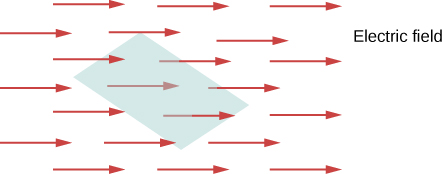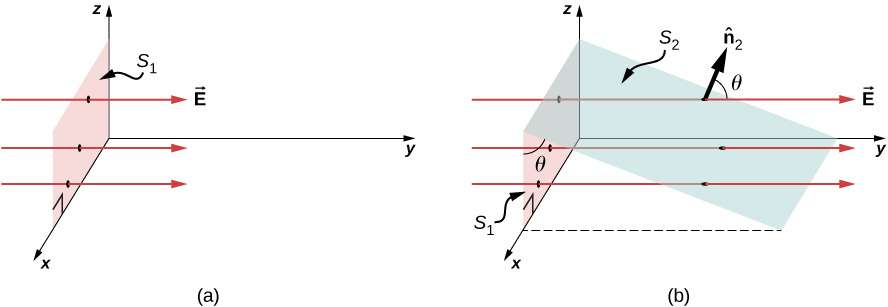# 6.1 Electric flux

 Page 1 / 8
By the end of this section, you will be able to:
• Define the concept of flux
• Describe electric flux
• Calculate electric flux for a given situation

The concept of flux    describes how much of something goes through a given area. More formally, it is the dot product of a vector field (in this chapter, the electric field) with an area. You may conceptualize the flux of an electric field as a measure of the number of electric field lines passing through an area ( [link] ). The larger the area, the more field lines go through it and, hence, the greater the flux; similarly, the stronger the electric field is (represented by a greater density of lines), the greater the flux. On the other hand, if the area rotated so that the plane is aligned with the field lines, none will pass through and there will be no flux.The flux of an electric field through the shaded area captures information about the “number” of electric field lines passing through the area. The numerical value of the electric flux depends on the magnitudes of the electric field and the area, as well as the relative orientation of the area with respect to the direction of the electric field.

A macroscopic analogy that might help you imagine this is to put a hula hoop in a flowing river. As you change the angle of the hoop relative to the direction of the current, more or less of the flow will go through the hoop. Similarly, the amount of flow through the hoop depends on the strength of the current and the size of the hoop. Again, flux is a general concept; we can also use it to describe the amount of sunlight hitting a solar panel or the amount of energy a telescope receives from a distant star, for example.

To quantify this idea, [link] (a) shows a planar surface ${S}_{1}$ of area ${A}_{1}$ that is perpendicular to the uniform electric field $\stackrel{\to }{\text{E}}=E\stackrel{^}{\text{y}}.$ If N field lines pass through ${S}_{1}$ , then we know from the definition of electric field lines ( Electric Charges and Fields ) that $N\text{/}{A}_{1}\propto E,$ or $N\propto E{A}_{1}.$

The quantity $E{A}_{1}$ is the electric flux    through ${S}_{1}$ . We represent the electric flux through an open surface like ${S}_{1}$ by the symbol $\text{Φ}$ . Electric flux is a scalar quantity and has an SI unit of newton-meters squared per coulomb ( $\text{N}·{\text{m}}^{2}\text{/C}$ ). Notice that $N\propto E{A}_{1}$ may also be written as $N\propto \text{Φ}$ , demonstrating that electric flux is a measure of the number of field lines crossing a surface .(a) A planar surface S 1 of area A 1 is perpendicular to the electric field E j ^ . N field lines cross surface S 1 . (b) A surface S 2 of area A 2 whose projection onto the xz -plane is S 1 .The same number of field lines cross each surface.

Now consider a planar surface that is not perpendicular to the field. How would we represent the electric flux? [link] (b) shows a surface ${S}_{2}$ of area ${A}_{2}$ that is inclined at an angle $\theta$ to the xz -plane and whose projection in that plane is ${S}_{1}$ (area ${A}_{1}$ ). The areas are related by ${A}_{2}\phantom{\rule{0.2em}{0ex}}\text{cos}\phantom{\rule{0.2em}{0ex}}\theta ={A}_{1}.$ Because the same number of field lines crosses both ${S}_{1}$ and ${S}_{2}$ , the fluxes through both surfaces must be the same. The flux through ${S}_{2}$ is therefore $\text{Φ}=E{A}_{1}=E{A}_{2}\phantom{\rule{0.2em}{0ex}}\text{cos}\phantom{\rule{0.2em}{0ex}}\theta .$ Designating ${\stackrel{^}{\text{n}}}_{2}$ as a unit vector normal to ${S}_{2}$ (see [link] (b)), we obtain

a diagram of an adult mosquito
what are white blood cells
white blood cell is part of the immune system. that help fight the infection.
MG
Mlungisi
Cells with a similar function, form a tissue. For example the nervous tissue is composed by cells:neurons and glia cells. Muscle tissue, is composed by different cells.
Anastasiya
I need further explanation coz celewi anything guys,,,
hey guys
Isala
on what?
Anastasiya
is air homogenous or hetrogenous
homogenous
Kevin
why saying homogenous?
Isala
explain if oxygen is necessary for photosynthesis
explain if oxygen is necessary for photosynthesis
Yes, the plant does need oxygen. The plant uses oxygen, water, light, and produced food. The plant use process called photosynthesis.
MG
By using the energy of sunlight, plants convert carbon dioxide and water into carbohydrates and oxygen by photosynthesis. This happens during the day and sunlight is needed.
NOBLE
no. it s a product of the process
Anastasiya
yet still is it needed?
NOBLE
no. The reaction is: 6CO2+6H20+ solar energy =C6H12O6(glucose)+602. The plant requires Carbon dioxyde, light, and water Only, and produces glucose and oxygen( which is a waste).
Anastasiya
what was the question
joining
Godfrey
the specific one
NOBLE
the study of non and living organism is called.
Godfrey
Is call biology
Alohan
yeah
NOBLE
yes
Usher
what Is ecology
what is a cell
A cell is a basic structure and functional unit of life
Ndongya
what is biolgy
is the study of living and non living organisms
Ahmed
may u draw the female organ
i dont understand
Asal
:/
Asal
me too
DAVID
anabolism and catabolism
Anabolism refers to the process in methabolism in which complex molecules are formed "built" and requires energy to happen. Catabolism is the opposite process: complex molecules are deconstructed releasing energy, such as during glicolysis.
Anastasiya
Explain briefly independent assortment gene .
hi
Amargo
hi I'm Anatalia
Joy
what do you mean by pituitary gland
Digambar
draw and lable the cell
why homones are destroyed soon after completing their role
Got questions? Join the online conversation and get instant answers!By OpenStaxBy OpenStaxBy David CoreyBy JavaChamp TeamBy OpenStaxBy OpenStaxBy RhodesBy OpenStaxBy Joanna SmithbackBy OpenStax